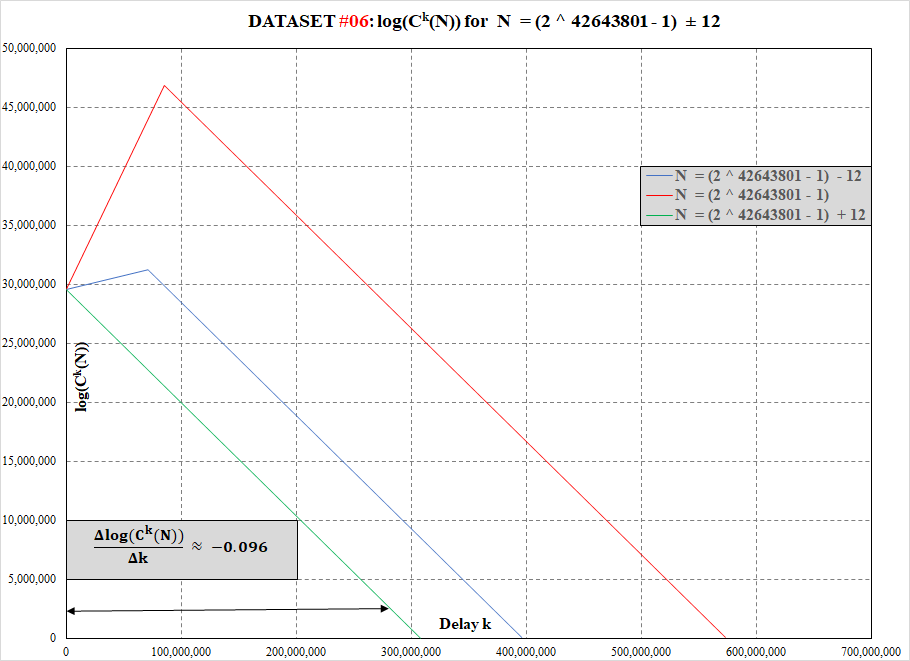# Collatz function data for 2^82589933 – 1 (#1 known megaprime)

We publish our first data on the Collatz function for 282589933 – 1  (#1 known megaprime) and two of its odd neighbors located at ±2.

# Collatz function data for 2^77232917 – 1 (#2 known megaprime)

We publish our first data on the Collatz function for 277232917 – 1  (#2 known megaprime) and two of its odd neighbors located at ±4.

# Collatz function data for 2^74207281 – 1 (#3 known megaprime)

We publish our first data on the Collatz function for 274207281 – 1  (#3 known megaprime) and two of its odd neighbors located at ±6.

# Collatz function data for 2^57885161 – 1 (#4 known megaprime)

We publish our first data on the Collatz function for 257885161 – 1  (#4 known megaprime) and two of its odd neighbors located at ±8.

# Collatz function data for 2^43112609 – 1 (#5 known megaprime)

We publish our first data on the Collatz function for 243112609 – 1  (#5 known megaprime) and two of its odd neighbors located at ±10.

# Additional Collatz function data for 2^42643801 – 1 (#6 known megaprime)

We publish further data on the Collatz function for 242643801 – 1  (#6 known megaprime) and two of its odd neighbors located at ±12.

Once the maximum is reached, the speed of decline for the log of the Collatz  function seems to be the same for all tested numbers and is equal to approximately -0.096.# Collatz function data for 2^42643801 – 1 (#6 known megaprime)

We publish our first data on the Collatz function for 242643801 – 1  (#6 known megaprime) and two of its odd neighbors located at ±12.

# Collatz function data for 2^37156667 – 1 (#7 known megaprime)

We publish our first data on the Collatz function for 237156667 – 1  (#7 known megaprime) and two of its odd neighbors located at ±26.

# Collatz function data for 2^32582657 – 1 (#8 known megaprime)

We publish our first data on the Collatz function for 232582657 – 1  (#8 known megaprime) and two of its odd neighbors located at ±71149323674102624414.

# Collatz function data for 10223×2^31172165 + 1 (#9 known megaprime)

We publish our first data on the Collatz function for 10223×231172165 + 1  (#9 known megaprime) and two of its odd neighbors located at ±4.

# Collatz function data for 2^30402457 – 1 (#10 known megaprime)

We publish our first data on the Collatz function for 230402457 – 1  (#10 known megaprime) and two of its odd neighbors located at ±2.

# Collatz function data for 2^25964951 – 1 (#11 known megaprime)

We publish our first data on the Collatz function for 2^25964951 – 1 (#11 known megaprime) and two of its odd neighbors located at ±2. Continue reading

# Collatz function data for 2^24036583 – 1 (#12 known megaprime)

We publish our first data on the Collatz function for 2^24036583 – 1 (#12 known megaprime) and two of its odd neighbors located at ±2. Continue reading

# Collatz function data for 2^20996011 – 1 (#13 known megaprime)

We publish our first data on the Collatz function for 2^20996011 – 1 (#13 known megaprime) and two of its odd neighbors located at ±2. Continue reading

# Collatz function data for 1059094^1048576 + 1 (#14 known megaprime)

We publish our first data on the Collatz function for 1059094^1048576 + 1 (#14 known megaprime) and two of its odd neighbors located at ±4. Continue reading